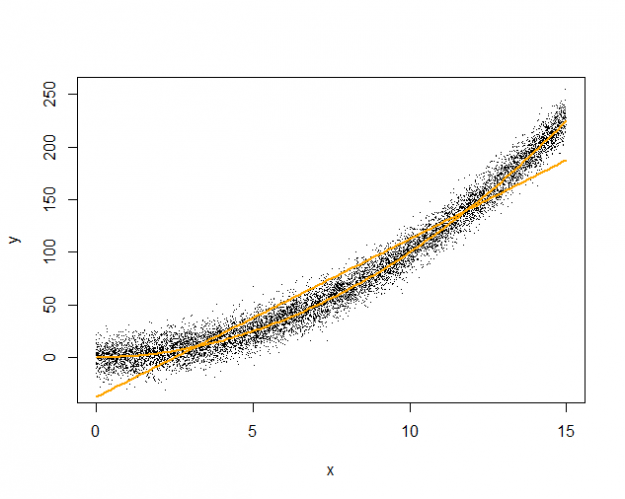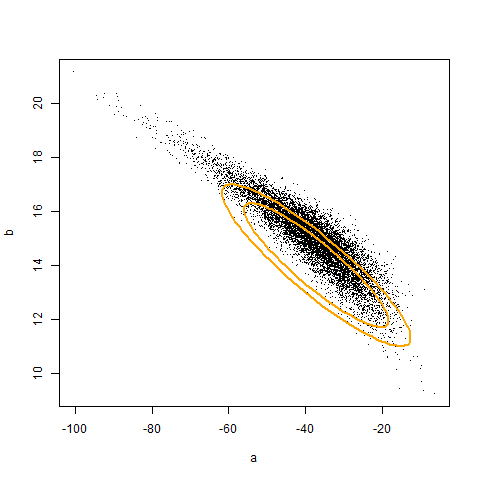# Making inferences about unusual population quantities.

This post builds on my last: Alternatives to model diagnostics for statistical inference?, where I had claimed that we could make quality inferences about the best linear approximation to a quadratic relationship. The R code below implements such a scenario, to establish a framework for discussion. I have inserted comments to augment the code.

```set.seed(42)
# Consider an infinite population where the association
# between two variables x and y is described by the following:
# y = x^2 + e
# x ~ U(0, 15)
# e ~ N(0, 10)

# We seek a linear approximation of the relationship between
# x and y that takes the form below, and minimizes the average
# squared deviation (i.e., the 'best' approximation).
# hat(y) = a + b*x

# This function simulates "n" observations from the population.
simulate <- function(n=20) {
x <- runif(n, 0, 15)
y <- rnorm(n, x^2, 10)
data.frame(y=y,x=x)
}

# This function finds the 'sample' best linear approximation to the
# relationship between x and y.
sam_fit <- function(dat)
lm(y ~ x, data=dat)

# We can approximate the 'population' best linear approximation by
# taking a very large sample. Note that this only works well for
# statistics that converge to a population quantity.
pop_fit <- sam_fit(simulate(1e6))

## > pop_fit
##
## Call:
## lm(formula = y ~ x, data = simulate(1e+06))
##
## Coefficients:
##   (Intercept)            x
##        -37.53        15.00
```

The figure below illustrates the true quadratic curve and the best linear approximation (pop_fit), overlaid against 10000 samples.```# This function creates a level confidence region for the intercept
# and slope of the best linear approximation, and tests whether
# the region includes the corresponding population values.
# confidence region larger or smaller.
sam_int <- function(dat, val=c(a=0, b=0), level=0.95, adj=0.00) {
s <- sam_fit(dat)
d <- coef(s) - val
v <- vcov(s)
as.numeric(d %*% solve(v) %*% d) < c
}

# By specifying that the region has 95% confidence, we intend that
# the region includes the population quantity in 95% of samples.
# We can assess the coverage of the above 95% confidence region by
# drawing repeated samples from the population and checking whether
# the associated confidence regions include the population values:
coverage20 <- mean(replicate(1e4, sam_int(simulate(20),
val=coef(pop_fit))))

## > coverage20
##  0.855

# Because the true coverage is less than the nominal coverage,
# the confidence region is anti-conservative. However, suppose that
# we can adjust the critical value of the region so that the true
# coverage is equal to the nominal value:
coverage20a <- mean(replicate(1e4, sam_int(simulate(20),
val=coef(pop_fit),